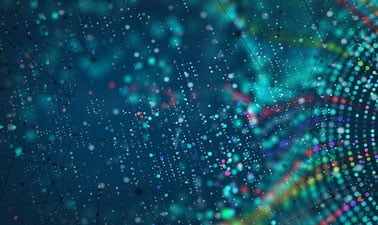# The Georgia Institute of Technology: Linear Algebra I: Linear Equations

4.0 stars
12 ratings

This course takes you through the first three weeks of MATH 1554, Linear Algebra, as taught in the School of Mathematics at The Georgia Institute of Technology.3 weeks
5–6 hours per week
Self-paced
Free

Systems of equations live at the heart of linear algebra. In this course you will explore fundamental concepts by exploring definitions and theorems that give a basis for this subject. At the start of this course we introduce systems of linear equations and a systematic method for solving them. This algorithm will be used for computations throughout the course as you investigate applications of linear algebra and more complex algorithms for analyzing them.

Later in this course you will later see how a system of linear equations can be represented in other ways, which can reduce problems involving linear combinations of vectors to approaches that involve systems of linear equations. Towards the end of the course we explore linear independence and linear transformations. They have an essential role throughout our course and in applications of linear algebra to many areas of industry, science, and engineering. __

### At a glance

• Institution: GTx
• Subject: Math
• Level: Intermediate
• Prerequisites:
None
• Language: English
• Video Transcript: English
• Associated programs:
• Associated skills: Linear Algebra, Linear Equations, Algorithms, Basic Math

# What you'll learn

Skip What you'll learn

Upon completion of this course, learners will be able to:

• Evaluate mathematical expressions to compute quantities that deal with linear systems
• Characterize a linear system in terms of the number of solutions, and whether the system is consistent or inconsistent.
• Apply elementary row operations to solve linear systems of equations.
• Characterize a set of vectors in terms of linear combinations, their span, and how they are related to each other geometrically
• Characterize a set of vectors and linear systems using the concept of linear independence.
• Construct dependence relations between linearly dependent vectors.
• Identify and construct linear transformations of a matrix.
• Characterize linear transformations as onto and/or one-to-one.